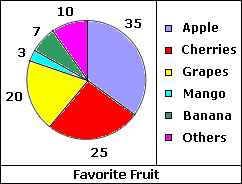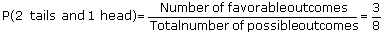Related Links

## Definition Of Statistics

Statistics is a branch of applied mathematics concerned with collecting, organizing, and interpreting data. The data are represented by means of graphs.

Statistics is also the mathematical study of the likelihood and probability of events occurring based on known quantitative data or a collection of data.

Statistics, thus attempts to infer the properties of a large collection of data from inspection of a sample of the collection thereby allowing educated guesses to be made with a minimum of expense.

There are basically three kinds of averages commonly used in statistics. They are: mean, median, and mode.

### Example of Statistics

A survey was conducted to find the favorite fruit of 100 people. The circle graph below shows the results of the survey.### Solved Example on Statistics

#### Ques: What is the probability of getting two tails and one head, when 3 coins are tossed at a time?

##### Choices:

A. 13
B. 14
C.3/8
D. 17
Correct Answer: C

### Solution:

Step 1: Number of possible outcomes when one coin is tossed = 2. [Outcomes are Head(H) and Tail(T).]
Step 2: The possible outcomes, when 3 coins are tossed are {TTT, THT, TTH, THH, HHT, HTH, HTT, HHH}.
Step 3: Number of favorable outcomes = 3 [Favorable outcomes are THT, TTH, HTT.]
Step 4:[Substitute.]
Step 5: So, probability of getting two tails and one head is 3/8.